## DEV Community is a community of 715,952 amazing developers

We're a place where coders share, stay up-to-date and grow their careers.

Eugenia

Posted on • Originally published at towardsdatascience.com on

# Linear and Bayesian modeling in R: Predicting movie popularity#### Which movie should I choose?

Let’s imagine a rainy day. You look outside through the window and everything is grey and cold. You grab your blanket and sit in your favourite couch; it is very cozy and comfy and you just decided it’s a perfect day to watch a movie. You want to watch a good one, or something at least that it is popular. You have several options, but some of them are not even rated in the typical movie websites! Wouldn’t be nice to be able to predict what people think of those movies? Well, maybe there is a solution for that. What about predicting a movie popularity according to some characteristics? We just need a dataset with movies, some statistical tools and R studio.

In our dataset, there is 651 randomly sampled movies which were released in United States movie theaters in the period of 1970–2016. The data was obtained from Rotten Tomatoes and IMDB. The dataset contains 32 features of each movie, including genre, MPAA rating, production studio, and whether they received Oscar nominations, among other characteristics. So now, we can ask ourselves:

Can the popularity of a movie be predicted by considering certain of its characteristics such as type, genre, MPAA rating, number of IMDb votes, and whether it has won an award?

Before going on developing any model, we need to answer two questions: Can our results be generalised? Which type of inference can be do with the present dataset?. For the first question, we can notice that the movies included in this dataset were randomly sampled from the above two mentioned sources and no bias was created by the sampling method, as a consequence, we can assume that the results obtained can be generalised to all U.S movies released between 1970 and 2016. On the other hand, this is an observational study, so the relationships that could be found from this data indicate association , but not causation.

Ready to start? Wait a second. What do we understand as “popularity” of a movie? Our dataset includes movies sample from two different sources and we have two variables that could potentially be used as popularity: audience_score(Audience score on Rotten Tomatoes) and imdb_rating(Rating on IMDB). So let’s go ahead and analyse a little more these two variables. First of all, we will check whether these variables show a correlation between them. For doing this, we will plot both variables in a scatter plot: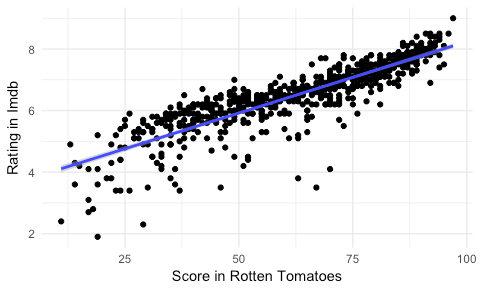We can see that the plot shows a possible positive correlation between the two variables. We will confirm this by using the function cor to numerically calculate the correlation:

As we can observed, there is a high correlation between both variables. If we are going to include one of the variables as response, it is better to not include the other one as independent variable. So we need to decide which one to select as response variable. We can analyse their distribution by making use of histogram and summary statistics to make a wise choice. Let’s start by imbd_rating: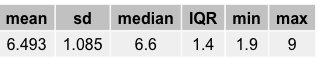Then, we can do the same for audience_score: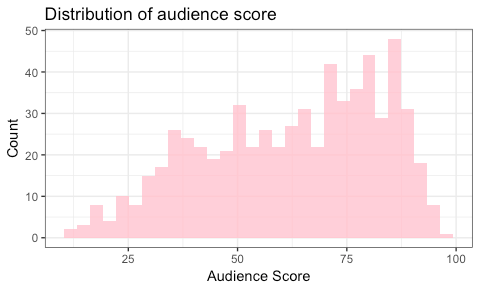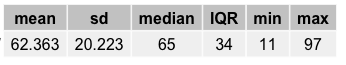We can see that the variable imbd_rating shows a distribution close to normal with a slightly left skew with a mean of 6.493 and a median of 6.00. On the other hand, the variable audience_score shows a more uniform distribution with a mean of 62.36 and a median of 65.00. Because of its distribution, we will choose to considered only imdb_rating.

After deciding which variable we will consider as our response variable, we need to explore our explanatory variables. We can analyse the distribution of the variables that we are interested in including in our model by plotting a histogram for each of the variables and obtaining summary descriptive tables. For those variables that are categorical , we can create a proportion table by using the build-in function table. On the other hand, we can create a data frame for continuous variables and apply the function summary. I will not show the entire code here, but for example, in our dataset, the analysis showed that the distribution of the number of votes was right skew. In order to adjust this, we can apply a log-transformation to the values (log_votes).

After that, we can analyse the interaction between our exploratory variables and the response variable. For this task, we can plot boxplot or scatter plots according to whether the exploratory variable is numerical o categorical. I will only show the significant findings.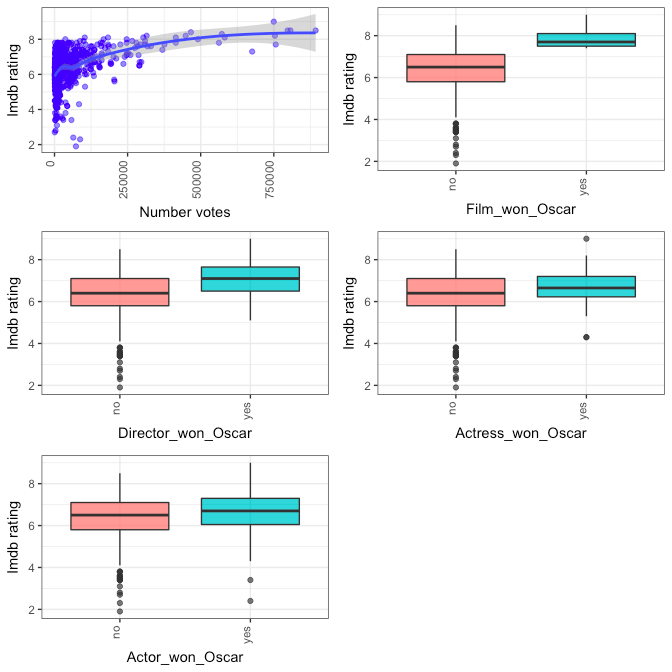From the plots and the summary descriptive obtained, it can be seen that, in our dataset, those movies that won an Oscar or the director ever won an Oscar appear to have a sightly higher rating. Moreover, the number of votes given show a weak positive association with the IMDB rating. Last, the variables best_actor_win and best_actress_win appear to have the same distribution and a similar association with imdb_rating, so we will combine these two variables in a new one called main_oscar_win.

Now, we have a good idea of what our response variable looks like and also a hint on which variables could be important to predict the popularity of a movie. It’s time to start building a model!. We will take two approaches here: first, we will do a multiple linear regression and then, we will develop a Bayesian model.

Multiple linear regression model

Multiple linear regression seek to model the relationship between two or more independent or explanatory variables and the response variable by fitting a linear equation to the data.

Our goal is to reach a parsimonious model , this is the simpler model with great explanatory predictive power. In order to do this, we have two options for model selection: Forward selection and backwards elimination. In the first case, we start with an empty model and we add one predictor at a time. We will choose the second option: Backwards elimination implies starting with a model comprising all candidates and dropping one predictor at a time until the parsimonious model is reached. In our case, our first full model will include six variables that we find before that could be important for predicting movie popularity: genre, best_pic_win, best_dir_win, main_oscar_win, log_votes and mpaa_rating. In R, we can use the function lm to build a linear model: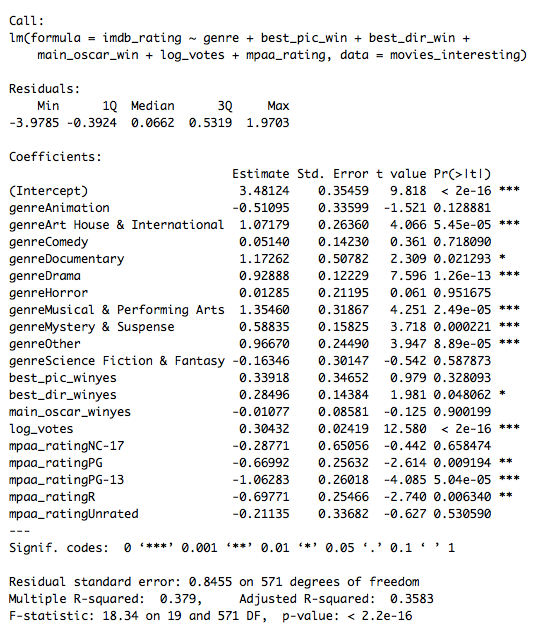Now that we have the full model, there are several criteria that we can use in order to drop variables: p-value and adjusted R². We will choose p-value as elimination criteria due to the fact that in this case, the aim is to create a model that shows the highest predictive value using only variables with significance.

After running the full model with all the variables involved, we have obtained an adjusted R² of 0.3582, which means that we can still improve the model. In order to do so, we can start by removing the variable which has the highest p-value each time, until all the variables remaining in the model are significant. So the variable that has the highest p-value in our model is main_oscar_win.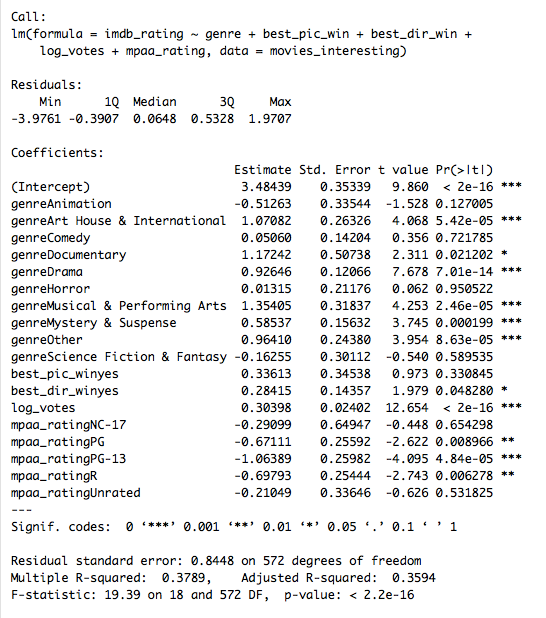After running again our simpler model, we can see that now our adjusted R² is 0.3594. We can try to improve our model more by eliminating again the variable with the highest p-value. In this case, it will be best_pic_win.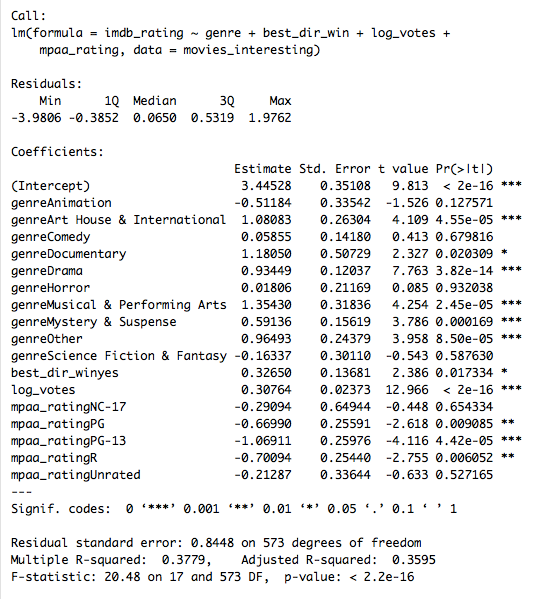We now see that the adjusted R² is 0.3595, not different from our previous model in step1, but with the difference that this time all variables involved are significant. I will not show it here for practical sake, but removal of any of other variables will decrease the adjusted R². So we considered this our final model.

There is a very important concept to have in mind for linear regression: Collinearity. Two variables are considered to be collinear when they are highly correlated with each other. The inclusion of collinear predictors complicates the model estimation.

So at this point, we can look into our variables and see if the variables we are interested in show some degree of collinearity. In our dataset, we have mixed variables, this is we have some variables that are categorical and some that are continuous, so in this case, a way to measure collinearity is using the variance inflation factor (VIF). The VIF, that quantifies the extent of multicollinearity in an ordinary linear regression, is calculated as the ratio between the variance of the model with multiple terms and the variance of the model with one term alone. In simple words, it tells us how much the variance of a regression coefficient increases due to collinearity existent in the model. So, let’s go ahead and calculate this: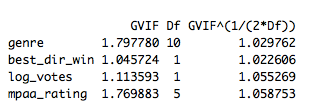None of our predictors has a high VIF, so we can assume that multicollinearity is not playing a role in our model.

Now, it’s time to run some diagnostic in our model. The multiple regression model depends on the following four assumptions:

1. Each numerical explanatory variable is linearly related to the response variable
2. Residuals are distributed nearly normal with a mean of 0
3. Variability of residuals is nearly constant
4. The residuals are independant

We will test one-by-one the assumptions in the context of our model:

1. The only numerical variable that we have in our model is log_values. So we can explore the first assumption by checking the residual plots.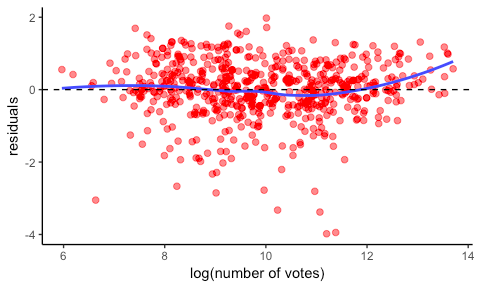The plot shows that the residuals are random scatter around 0, which indicates a linear relationship between the numerical exploratory variable and the response variable.

1. To check this condition, we will perform first the histogram of the residuals and then a residuals Q-Q plot.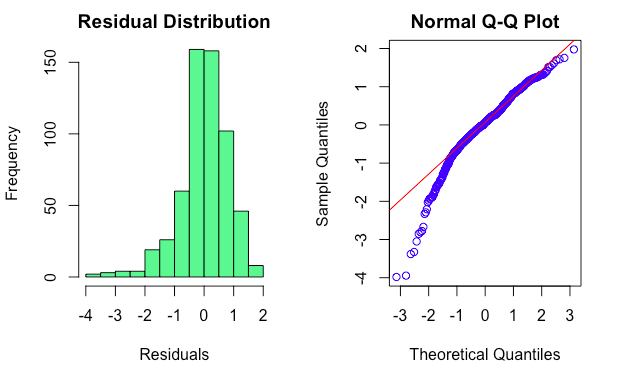As we can see above, the distribution histogram and the residuals Q-Q plot show a close to normal distribution, and also mimics the left-hand skew that was observed in the original imdb rating variable.

1. Now, we need to check that the residuals are equally variable for low and high values of the predicted response variable. Then, we will check the plot of residuals vs. predicted.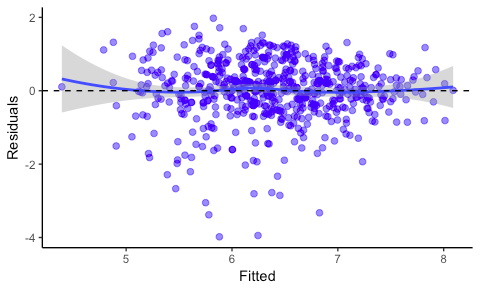The residuals are randomly scattered in a band with a constant width around 0.

1. Lastly, we will check for the independency of the residuals: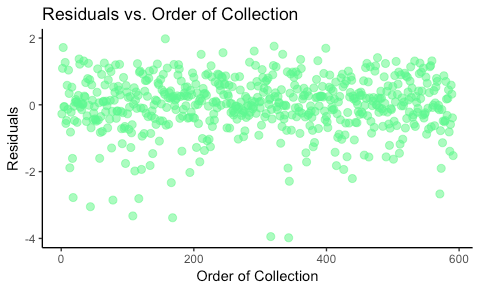The plot above does not display any particulat pattern, so it is possible to assume that the residuals and as a consequence, the observations are independant.

Bayesian model

Usually, we are taught traditional frequentist statistics to solve a problem. However, there is another approach which it is sometimes undermine for being subjective, but which is more intuitive or close to how we think about probability in everyday life and yet is a very powerful tool: Bayesian statistics. There are some key concept on which this theory relies: Conditional probability and Bayes theorem.

Conditional probability i_s the probability that an event will happen given that another event took place. If the event B is known or assumed to have taken place, then the conditional probability of our event of interest A given B is written as _P(A|B).

When two events are independent, meaning that A happening is not affecting B to happen, the conjunction probability of A and B (in other words, the probability of both events being true) is written as P(A and B)= P(A) P(B). But this is not the case if A conditions B to happen, where the conjunction probability is p(A and B) = p(A) p(B|A).

Here, after some mathematical calculations, the Bayes theorem can be derived and it is presented as follows:

p(A|B) = p(A) p(B|A) / p(B)

To put this on words: the probability of A given that B have occurred is calculated as the unconditioned probability of A occurring multiplied by the probability of B occurring if A happened, divided by the unconditioned probability of B.

There is a powerful interpretation of this theorem called diachronic interpretation , meaning that something is happening over time, and it gives a tool to update the probability of a hypothesis provided new data. In this interpretation, the terms in our equations implies some other concepts:

• p(A) is the probability of the hypothesis before we see the data, called the prior probability, or just prior.
• p(A|B) is our goal, this is the probability of the hypothesis after we see the data, called the posterior.
• p(B|A) is the probability of the data under the hypothesis, called the likelihood.
• p(B) is the probability of the data under any hypothesis, called the normalizing constant.

There is an element which is key when we want to build a model under Bayesian approach: the Bayes factor. The Bayes factor is the ratio of the likelihood probability of two competing hypotheses (usually null and alternative hypothesis) and it helps us to quantify the support of a model over another one. In Bayesian modelling, the choice of prior distribution is a key component of the analysis and can modify our results; however, the prior starts to lose weight when we add more data. Non informative priors are convenient when the analyst does not have much prior information.

In R, we can conduct Bayesian regression using the BAS package. We will use Bayesian Model Averaging ( BMA ), that provides a mechanism for accounting for model uncertainty, and we need to indicate the function some parameters:

Prior: Zellner-Siow Cauchy (Uses a Cauchy distribution that is extended for multivariate cases)

Model prior: Uniform (assign equal probabilities to all models)

Method: Markov chain Monte Carlo ( MCMC )( improves the model search efficiency)

We will now print the marginal inclusion probabilities obtained for the model: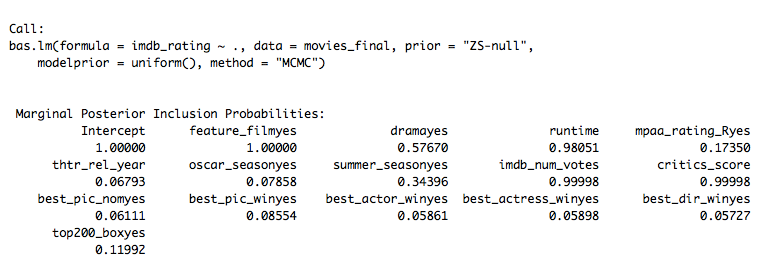After that, we can use the function summary to see the top 5 models with the zero-one indicators for variable inclusion.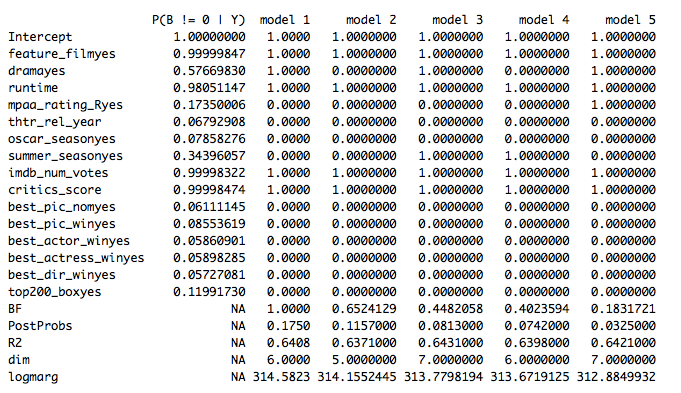It is also displayed a column with the Bayes factor (BF) for each model to the highest probability model, the posterior probabilities of the models (PostProbs), the R² of the models, the dimension of the models (dim) and the log marginal likelihood (logmarg) under the selected prior distribution.

Last, we can make use of the function image to visualize the Log Posterior Odds and Model Rank.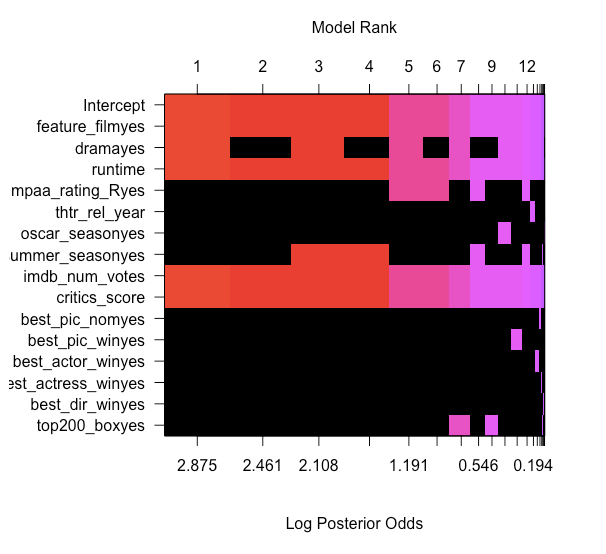In the picture above, each row correspond to each variable included in the full model as well as one extra row for the intercept. In each column, we can see all possible models (2¹⁶ because we have 16 variables included) sorted by their posterior probability from the best to worst rank on the top (from left to right).

From the model and the image above, we can see that:

> feature_film has a marginal probability of 0.999, and appears in all five top models

> critics_score has a marginal probability of 0.999 and also appears in all five top models

> runtime has a marginal probability of 0.98 and appears in all five top models

> drama has a marginal probability of 0.57 and appears in three of the five top models

> imbd_num_votes has a marginal probability of 0.99 and appears in three of the five top models

> the intercept also has a marginal probability of 1, and appears in all five top models

According to this, the best model includes the intercept, feature_film, critics_score, drama, imbd_num_votes and runtime

We can now obtain the coefficients estimates and standard deviations under BMA in order to be able to examine the marginal distributions for the important variables coefficients. To do so, we will use the function coef and plot them using plot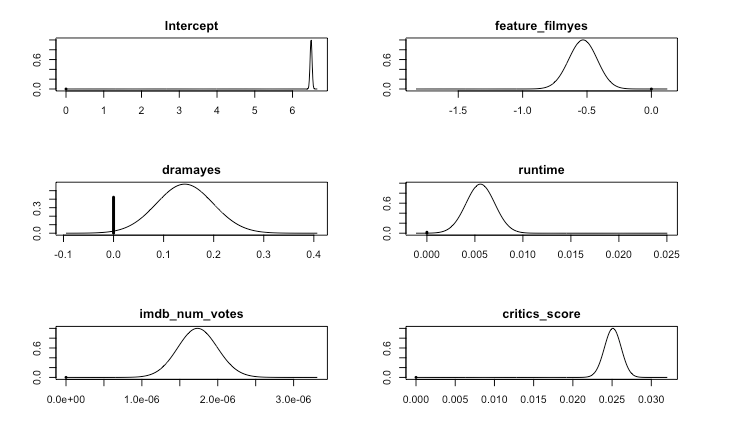The vertical line corresponds to the posterior probability that the coefficient equals to 0. On the other hand, the shaped curve shows the density of posiible values where the coefficient is non-zero. It is worthy to mention that the height of the line is scaled to its probability. This implies that intercept and feature_film, critics_score, imbd_num_votes and runtime show no line denoting non-zero probability.

Last, we can obtain 95% credible intervals (The probability that the true mean is contained within a given interval is 0.95) for coefficients using confint method.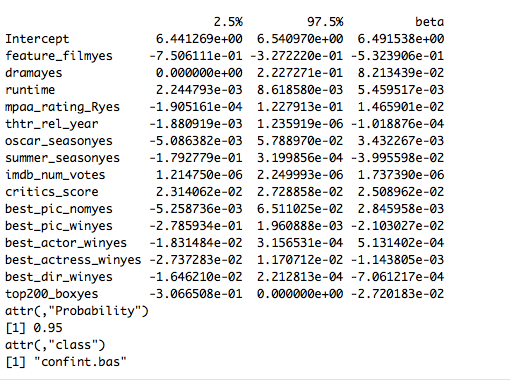BAS package provides us with an easy way to get graphical summaries for our model just using the function plot and the which option.

1. Residual vs. fitted plot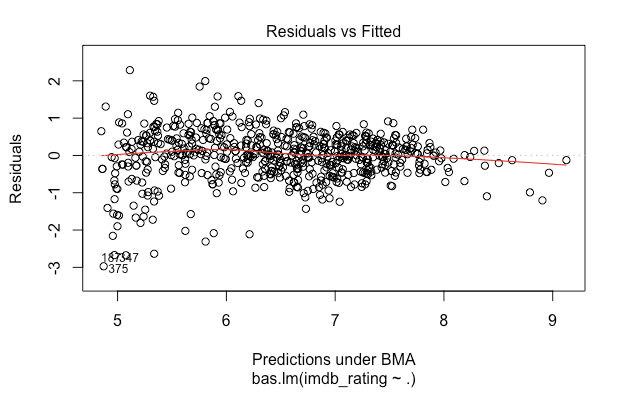Ideally, we will expect to not see outliers or non-constant variance. However, in this case we can see that there is a constant spread over the prediction but there are two outliers.

1. Model probabilities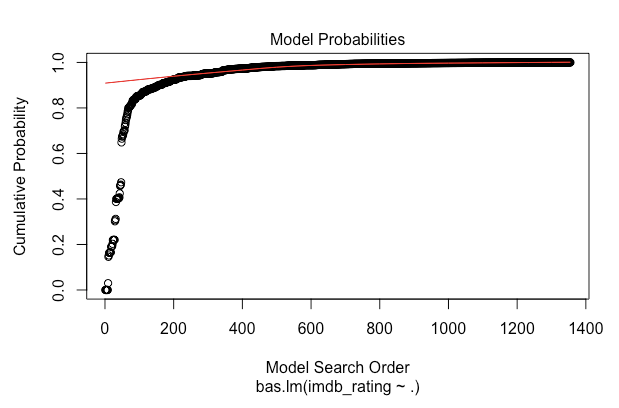This plot displays the cumulative probability of the models in the order that they are sampled. This plot shows that the cumulative probability starts to level off after 300 model trials as each additional model adds only a small increment to the cumulative probability. The model search stops at ~1400 instead of enumerations of 2¹⁵ combinations.

1. Model complexity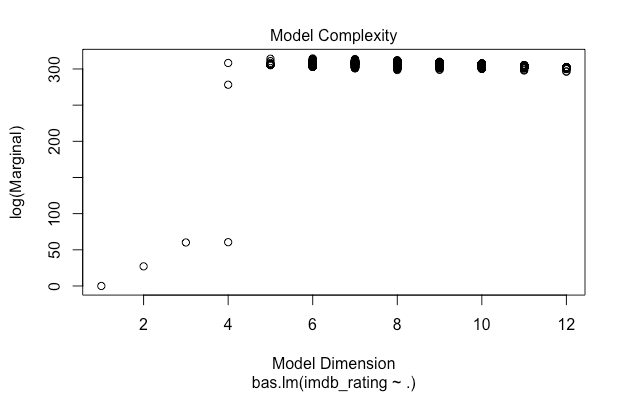This plot shows the dimension of each model, that is the number of regression coefficients including the intercept versus the log of the marginal likelihood of the model. In this case, we can see that highest log marginal can be reached from 5 to 12 dimensions.

1. Marginal inclusion probabilities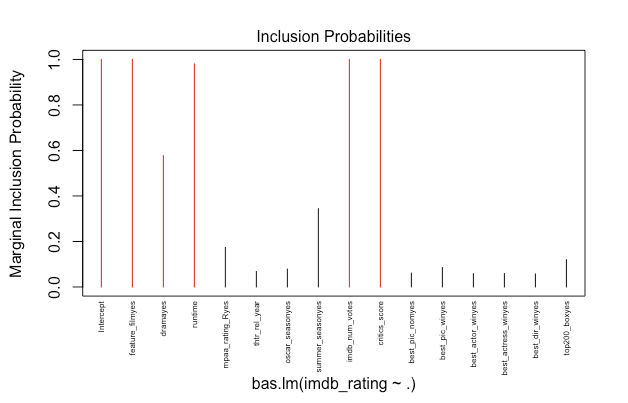In this case, we can observe the marginal posterior inclusion probabilities for each of the covariates, with marginal posterior inclusion probabilities that are greater than 0.5 shown in red (important variables for explaining the data and prediction). In the graph, we can see what it was show already before about which variables contribute to the final scores.

PREDICTION

Now it’s time to test the predictive capability of our two models! We will use the movie “Zootropolis” released in 2016. The corresponding information was obtained from the IMDB website and RottenTomatoes to be consistent with the analysis data.As we saw above, the true imdb_rating is 8, which is pretty close to what our Bayesian model predicted.

So what can we conclude? From the linear regression and the Bayesian model we learnt that in fact the popularity of a movie can be predicted by considering characteristic data of each movie.

In the linear regression analysis, it was possible to build a parsimonious, multivariable, linear model that is able to some extend to predict the movie popularity, understood as IMDb rating, with the four statistically significant predictors chosen. However, it is important to remember that the adjusted R² of our final model is only 0.3595, so this means that 35.95% of the variability is explained by the model. In the Bayesian model, we finally got a parsimonious model that also fullfilled the Bayesian assumptions.

From both models, we can see that the Bayesian model is the one which prediction was close to the real IMDb rating.

References:

• Peng Roger D. (2016) _Exploratory Data Analysis with R. _LeanPub
• Downey Allen B. (2012) Think Bayes. Bayesian Statistics in Python. Green Tea Press.

## Discussion (1)Uttasarga Singh

Hello Eugenia,

Thank you very much for such a splendid documentation. I really enjoyed the detail answers and statistical knowledge behind the same.
With that being said, suppose I have a list of movies for which I want to predict(say a million records), I don't want to type for each and every movie; the corresponding parameters, Is there a possibility that I can predict those using my model which I build by a single line of Code?